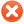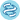Plot options# Use multiple genesUse list of genes Use mean expression of selected genes Use ratio of two genes Filter by median expression
more genes param

# ExampleExample input for multiple genes:
1007_s_at 1
200000_s_at -1
31637_s_at 0.5
40016_g_at -2

Explanation:
first: normal,
second: gene is inverted,
third: gene has weight,
fourth: gene has weight and is inverted

You can also use following format:
1007_s_at, 1
200000_s_at, -1
31637_s_at, 0.5
40016_g_at, -2

 Split patients by --none--lower quartilelower tertilemedianupper tertileupper quartile Auto select best cutoffTrichotomization : --none--T1 vs T3Q1 vs Q4Survival: PFS (n=1436)OS (n=1657)PPS (n=782)Compute median survival:Follow up threshold: all2 months4 months6 months12 months18 months24 months30 months36 months48 months60 months120 months180 months240 monthsCensore at threshold:## Probe set options

Use
 user selected probe set all probe sets per geneonly JetSet best probe setPlot beeswarm graph of probe distribution:## Restrict analysis to subtypes...

 Histology: all Endometrioid (n=62) Serous (n=1232) Stage: all 1 (n=107) 1+2 (n=179) 2 (n=72) 2+3 (n=1151) 2+3+4 (n=1340) 3 (n=1079) 3+4 (n=1268) 4 (n=189) Grade: all 1 (n=56) 1+2 (n=381) 2 (n=325) 2+3 (n=1349) 3 (n=1024) 4 (n=21) TP53 mutation: all mutated (n=516) wild type (n=102) Average CA125 below lower quartile:## Restrict analysis to treatment groups...

 Debulk: all optimal (n=802) suboptimal (n=536) Chemotherapy:all patients contains platin (n=1438) contains Taxol (n=821) contains Taxol + platin (n=804) contains Avastin (n=50) contains Docetaxel (n=108) contains Gemcitabine (n=135) contains Paclitaxel (n=248) contains Topotecan (n=119)

## Use following dataset(s) for the analysis:

 alleach separatelyGSE14764 (n=80)GSE15622 (n=35)GSE18520 (n=63)GSE19829 (n=28)GSE23554 (n=28)GSE26193 (n=107)GSE26712 (n=195)GSE27651 (n=49)GSE30161 (n=58)GSE3149 (n=116)GSE51373 (n=28)GSE63885 (n=101)GSE65986 (n=55)GSE9891 (n=285)TCGA (n=565) (GSE14764:)

Array quality control:Please note: the generated p value does not include correction for multiple hypothesis testing by default.Please kindly cite following paper to support further development: Gyorffy B: Discovery and ranking of the most robust prognostic biomarkers in serous ovarian cancer, Geroscience, 2023, doi: 10.1007/s11357-023-00742-4.